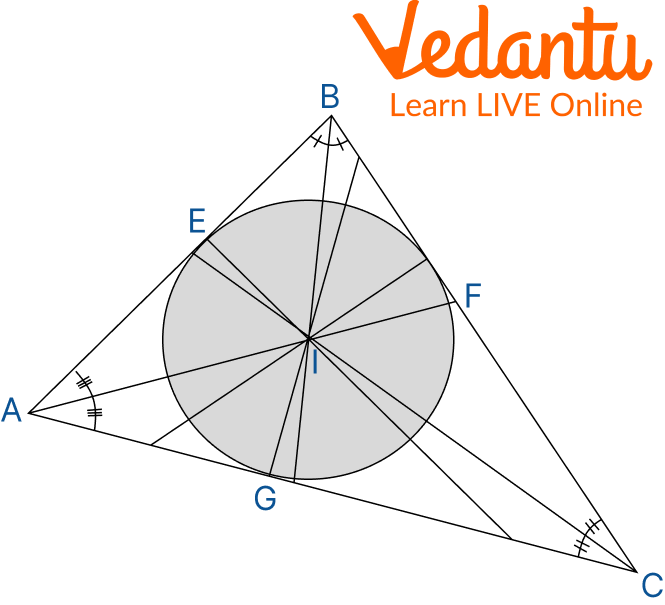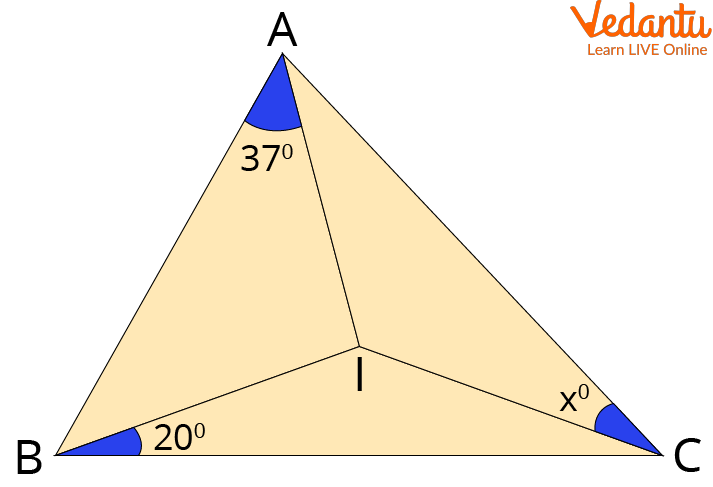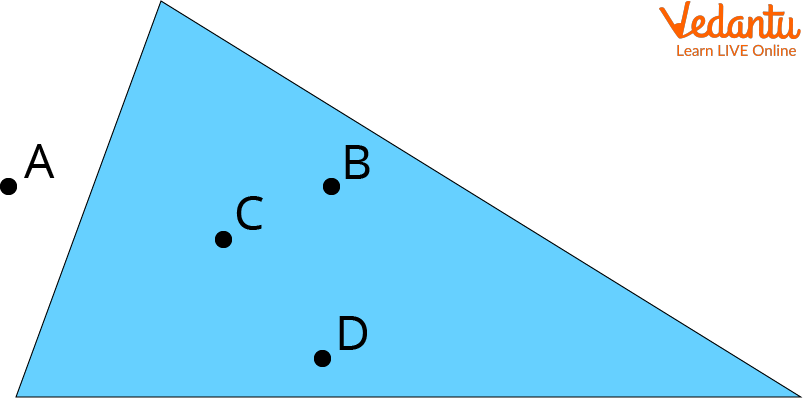Courses
Courses for Kids
Free study material
Free LIVE classes
More

# Incircle of a TriangleLIVE
Join Vedantu’s FREE Mastercalss

## What is a Triangle?

A triangle in geometry is a three-sided polygon with three edges and three vertices. The fact that a triangle's interior angles add up to 180 degrees is its most crucial characteristic. This characteristic is known as the triangle's angle sum property. If ABC is a triangle, it is written as ABC, where A, B, and C represent the triangle's vertices.

In Euclidean geometry, a triangle is a two-dimensional shape that is represented by three non-collinear points in a single plane. A triangle is a form of a polygon with three sides; the intersection of the two longest sides is known as the triangle's vertex.

## Have a look at Incircle of a Triangle

The greatest circle that may fit within a triangle in geometry is known as the incircle or inscribed circle, which touches (or is tangent to) all three sides of the triangle. The triangle's incenter, also known as the triangle's centre, sits at the centre of the incircle. The intersection of the three internal angle bisectors, or the incenter, marks the location of the incircle's centre.

## Properties of Incentre

### There are several characteristics of a triangle's incenter; let's examine the image below and list each one one by one.Incentre

Property 1: Line segments AE and AG, CG and CF, BF and BE are equal in length if I is the triangle's incenter.

Proof: As triangles, AEI and AGI are congruent triangles according to the rule of congruence.

Here AI = AI is common

The radius of the circle is IE = IGAnd $90^{\circ}$ angles are $\angle A E I=\angle A G I=90^{\circ}$

Thus, $\Delta AEI\cong \Delta AGI$

So, By CPCT we have AE = AG similarly which implies CG = CF AND BF = BE

Property 2: $\angle \text{BAl}=\angle \text{CAl},\angle \text{ABI}=\angle \text{CBI, }\angle \text{BCl}=\angle \text{ACl}$ if I is the triangle’s incentre.

### Proof: According to the rule of congruence by RHS we have AEI and AGI congruent triangles.

Thus, by CPCT, angle BAI is equal to angle CAI.

Property 3: The triangle's sides are tangents to the circle and are referred to as the circle's inradii. Here, OE = OF = OG = r are the inradii.

Property 4: We have $s=\dfrac{a+b+c}{2}$ where ‘s’ is semi perimeter, ‘r’ is inradius then area is A = sr

Property 5: A triangle's incenter, as opposed to an orthocenter, always resides within the triangle.

## Incircle of Triangle Formula

Here let E, F, and G be the angle bisectors of C, A, and B which cross the sides AB, AC, and BC respectively.

Now, use the angle sum property for calculating the incentre of a triangle angle.

Thus, we have $\angle AIB=180{}^\circ -\left( \dfrac{\angle A+\angle B}{2} \right)$ where ‘I’ is the incentive.

If we want to calculate it by using three coordinates. Coordinates are

$A{{(x)}_{1}},{{(y)}_{1}},B{{(x)}_{2}},{{(y)}_{2}},C{{(x)}_{3}},{{(y)}_{3}}$ for sides a, b, c.

Thus, incentre formula be $\left( \dfrac{a{{x}_{1}}+b{{x}_{2}}+c{{x}_{3}}}{a+b+c},\dfrac{a{{y}_{1}}+b{{y}_{2}}+c{{y}_{3}}}{a+b+c} \right)$

## Solved Examples

Q 1. ‘I’ be the incentre of the triangle in ABC triangle, find the value of ‘x’

Ans. Given that ‘I’ is the incenter.

According to the figure, we have AI, BI, and CI as angle bisectors.Triangle Showing Incentre

$\angle \text{BAl}+\angle \text{CBI}+\angle \text{ACl }=\dfrac{{{180}^{{}^\circ }}}{2}$

${{37}^{{}^\circ }}+{{20}^{{}^\circ }}+{{x}^{{}^\circ }}={{90}^{{}^\circ }}$

${{57}^{{}^\circ }}+{{x}^{{}^\circ }}={{90}^{{}^\circ }}$

${{x}^{{}^\circ }}={{90}^{{}^\circ }}-{{57}^{{}^\circ }}$

${{x}^{{}^\circ }}={{33}^{{}^\circ }}$

Q 2. Sandeep determined that a triangular sheet has a surface area of 50 feet square. The sheet has a 10-foot radius. Please assist Ram with determining the triangle's semiperimeter if a circle is drawn inside the triangle, touching each side.

Ans. We have given that the area is 50 feet square and the radius is 10 feet.

Area = semiperimeter which is multiplied by the inradius of the triangle.

$50=s\times 10$

$s=\dfrac{50}{10}$

$s=5$

## Practice Problems

Q 1. The triangle ABC constructed by the points A(3, 1), B(0, 3), and C(-3, 1) has the following coordinates for its incenter: (p, q). Find (p, q).

Ans: Coordinates = $\left( 0,\dfrac{2\sqrt{13}+18}{6+2\sqrt{13}} \right)$

Q 2. ‘I’ be the incentre of the triangle in PQR triangle, solve for the value of ‘x’ where $P=40{}^\circ , Q=20{}^\circ$

Ans: $x{}^\circ =30{}^\circ$

Q 3. Whichever of the following is true for the triangle's incenter.

1. The place where the triangle's perpendicular bisectors intersect is known as the incenter.

2. One of a triangle's sides may be where the triangle's incenter is located.

3. A triangle's incenter is located at equal distances from its vertices.

4. None of the above

Ans: Option D i.e. none of the above

Q 4. What is the incentre in the given figureIncentre

Ans: Here, ‘C’ is the incentre in the above figure.

## Summary

This article summarizes that a triangle in geometry is a three-sided closed polygon with three edges and three vertices and the greatest circle that may fit within a triangle in geometry is known as the incircle or inscribed circle, which touches (or is tangent to) all three sides of the triangle. The incenter of a triangle is defined as the point at which all three interior angle bisectors intersect. The properties of the Incenter and some solved examples on incenter are also discussed in this article which helps you to get a better understanding of the concept of Incircle.

Last updated date: 27th Sep 2023
Total views: 97.5k
Views today: 1.97k

## FAQs on Incircle of a Triangle

1. What is the importance of the incenter?

The incenter, also known as the centre of the inscribed circle, is the location where three of a triangle's interior angle bisectors connect. It has several important properties and relationships with other parts of the triangle, including its circumcenter, orthocenter, area, and others. A clock could be constructed using the incenter. To locate the centre of the circle, you wouldn't want the clock's hands to be off-centre.

2. The definitions of incircle and circumcircle

Every point in a circle is located at the same distance from a fixed point. A circle that has been drawn around the specified polygon is called a circumcircle. An incircle is a circle that is drawn inside a polygon and contacts all of its sides. A triangle's circumcircle is a circle that goes through each of its three vertices (polygon). The junction of the perpendicular bisectors of the triangle's sides marks the centre of the circle (polygon).

3. What is the incircle's mechanism?

A circle that is both inside the figure and perpendicular to each side is called an incircle of a convex polygon. Every triangle and regular polygon has a distinct incircle, although non-square rectangles and other polygons with four or more sides typically don't. Tangential quadrilaterals are quadrilaterals that don't have an incircle.

4. Is the centre of a triangle always inside?

It is the location of the circle's origin that is encircled by a triangle. The incenter always lies inside the triangle, much like the centroid. It is created by taking the point where the angle bisectors connecting the triangle's three vertices intersect.